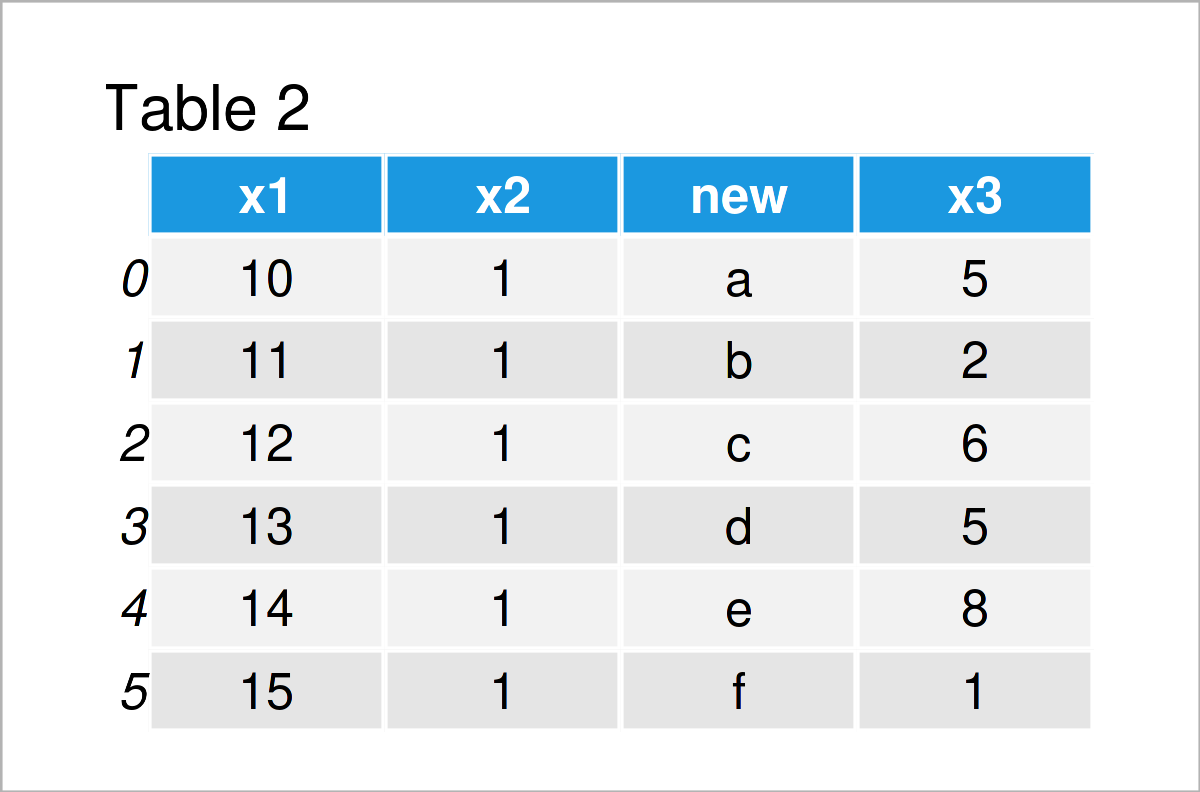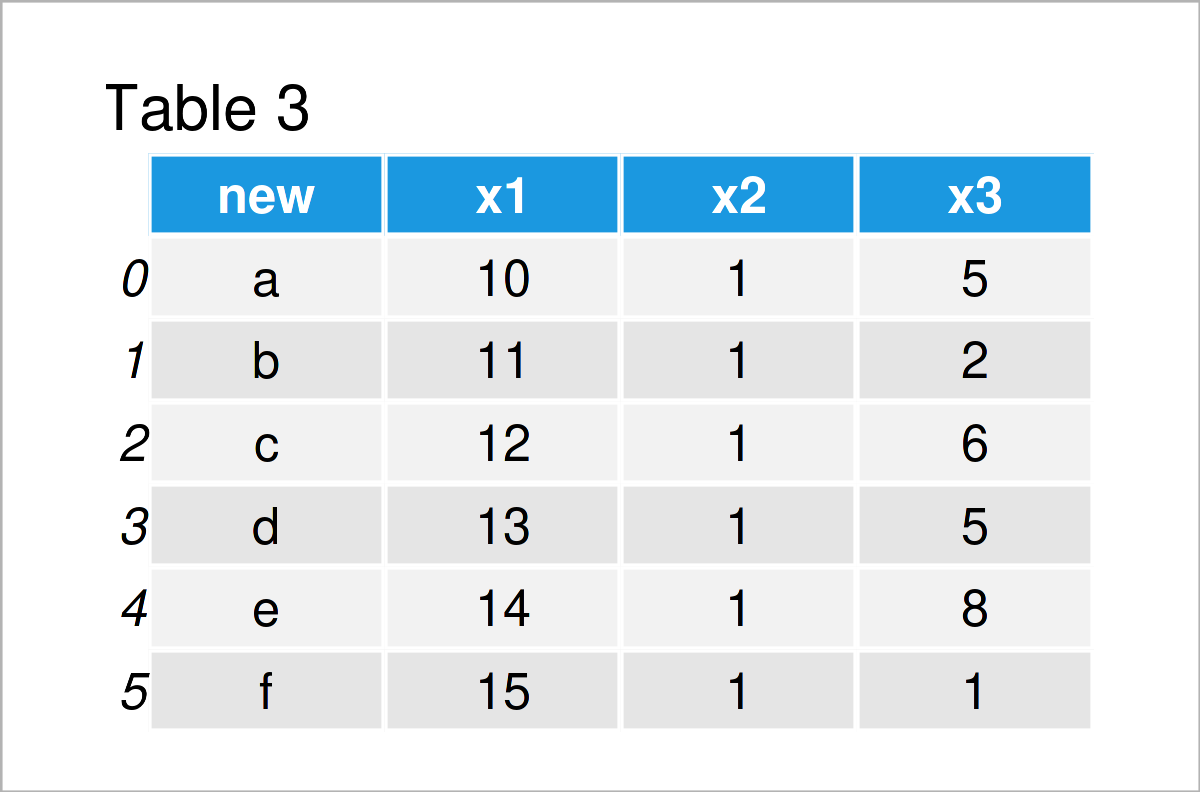# Insert Column at Specific Position of pandas DataFrame in Python (2 Examples)

In this Python tutorial you’ll learn how to add a column at a certain location of a pandas DataFrame.

The article will consist of these topics:

Here’s how to do it…

## Exemplifying Data & Software Libraries

First, we have to import the pandas library:

`import pandas as pd # Import pandas library`

Next, let’s also create some example data:

```data = pd.DataFrame({'x1':range(10, 16), # Create example DataFrame 'x2':[1, 1, 1, 1, 1, 1], 'x3':[5, 2, 6, 5, 8, 1]}) print(data) # Print example DataFrame```Have a look at the table that has been returned after running the previous syntax. It shows that our example data consists of six rows and the three columns “x1”, “x2”, and “x3”.

In addition, we have to create a list that we can add as a new column to our data set.

```new_col = ['a', 'b', 'c', 'd', 'e', 'f'] # Create example list print(new_col) # Print example list # ['a', 'b', 'c', 'd', 'e', 'f']```

As you can see, our list contains six different alphabetical letters.

## Example 1: Insert New Column in the Middle of pandas DataFrame

The Python code below illustrates how to insert a list as a new variable in between a pandas DataFrame.

For this task, we can apply the insert function as shown below. Within the insert function, we have to specify the index location of the new column, the name of the new column, as well as the value of the new column (i.e. our list).

```data_new1 = data.copy() # Create duplicate of data data_new1.insert(loc = 2, column = 'new', value = new_col) # Insert column print(data_new1) # Print updated data```After executing the previous Python syntax the new pandas DataFrame shown in Table 2 has been created. As you can see, we have inserted a new column in the middle of our data matrix.

## Example 2: Insert New Column at the Beginning of pandas DataFrame

This example illustrates how to append a new column at the very beginning of a pandas DataFrame.

For this task, we simply have to set the loc argument within the insert function to be equal to 0:

```data_new2 = data.copy() # Create duplicate of data data_new2.insert(loc = 0, column = 'new', value = new_col) # Add column print(data_new2) # Print updated data```In Table 3 you can see that we have created another pandas DataFrame with a new column at the first position of our data using the previous Python syntax.

## Video, Further Resources & Summary

Do you need further explanations on the Python code of this tutorial? Then you may have a look at the following video on the YouTube channel of Corey Schafer. In the video, he illustrates how to add and remove rows and columns to a pandas DataFrame using the Python programming language:

Please accept YouTube cookies to play this video. By accepting you will be accessing content from YouTube, a service provided by an external third party.If you accept this notice, your choice will be saved and the page will refresh.

Besides the video, you might want to read some of the other tutorials on Statistics Globe:

On this page you have learned how to append a variable at a certain location of a pandas DataFrame in the Python programming language. If you have additional questions, let me know in the comments.

Subscribe to the Statistics Globe Newsletter